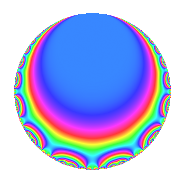# Properties

 Label 3969.1.t.cLevel $3969$ Weight $1$ Character orbit 3969.t Analytic conductor $1.981$ Analytic rank $0$ Dimension $2$ Projective image $D_{2}$ CM/RM discs -3, -7, 21 Inner twists $8$

# Related objects

## Newspace parameters

 Level: $$N$$ $$=$$ $$3969 = 3^{4} \cdot 7^{2}$$ Weight: $$k$$ $$=$$ $$1$$ Character orbit: $$[\chi]$$ $$=$$ 3969.t (of order $$6$$, degree $$2$$, not minimal)

## Newform invariants

 Self dual: no Analytic conductor: $$1.98078903514$$ Analytic rank: $$0$$ Dimension: $$2$$ Coefficient field: $$\Q(\zeta_{6})$$ Defining polynomial: $$x^{2} - x + 1$$ Coefficient ring: $$\Z[a_1, \ldots, a_{25}]$$ Coefficient ring index: $$1$$ Twist minimal: no (minimal twist has level 63) Projective image $$D_{2}$$ Projective field Galois closure of $$\Q(\sqrt{-3}, \sqrt{-7})$$ Artin image $C_3\times D_4$ Artin field Galois closure of $$\mathbb{Q}[x]/(x^{12} - \cdots)$$

## $q$-expansion

The $$q$$-expansion and trace form are shown below.

 $$f(q)$$ $$=$$ $$q - q^{4} +O(q^{10})$$ $$q - q^{4} + q^{16} + \zeta_{6}^{2} q^{25} -2 \zeta_{6}^{2} q^{37} + 2 \zeta_{6} q^{43} - q^{64} + 2 q^{67} + 2 q^{79} +O(q^{100})$$ $$\operatorname{Tr}(f)(q)$$ $$=$$ $$2q - 2q^{4} + O(q^{10})$$ $$2q - 2q^{4} + 2q^{16} - q^{25} + 2q^{37} + 2q^{43} - 2q^{64} + 4q^{67} + 4q^{79} + O(q^{100})$$

## Character values

We give the values of $$\chi$$ on generators for $$\left(\mathbb{Z}/3969\mathbb{Z}\right)^\times$$.

 $$n$$ $$2108$$ $$3727$$ $$\chi(n)$$ $$\zeta_{6}^{2}$$ $$-\zeta_{6}^{2}$$

## Embeddings

For each embedding $$\iota_m$$ of the coefficient field, the values $$\iota_m(a_n)$$ are shown below.

For more information on an embedded modular form you can click on its label.

Label $$\iota_m(\nu)$$ $$a_{2}$$ $$a_{3}$$ $$a_{4}$$ $$a_{5}$$ $$a_{6}$$ $$a_{7}$$ $$a_{8}$$ $$a_{9}$$ $$a_{10}$$
2971.1
 0.5 − 0.866025i 0.5 + 0.866025i
0 0 −1.00000 0 0 0 0 0 0
3106.1 0 0 −1.00000 0 0 0 0 0 0
 $$n$$: e.g. 2-40 or 990-1000 Significant digits: Format: Complex embeddings Normalized embeddings Satake parameters Satake angles

## Inner twists

Char Parity Ord Mult Type
1.a even 1 1 trivial
3.b odd 2 1 CM by $$\Q(\sqrt{-3})$$
7.b odd 2 1 CM by $$\Q(\sqrt{-7})$$
21.c even 2 1 RM by $$\Q(\sqrt{21})$$
63.h even 3 1 inner
63.i even 6 1 inner
63.j odd 6 1 inner
63.t odd 6 1 inner

## Twists

By twisting character orbit
Char Parity Ord Mult Type Twist Min Dim
1.a even 1 1 trivial 3969.1.t.c 2
3.b odd 2 1 CM 3969.1.t.c 2
7.b odd 2 1 CM 3969.1.t.c 2
7.c even 3 1 567.1.l.b 2
7.c even 3 1 3969.1.k.b 2
7.d odd 6 1 567.1.l.b 2
7.d odd 6 1 3969.1.k.b 2
9.c even 3 1 441.1.m.a 2
9.c even 3 1 3969.1.k.b 2
9.d odd 6 1 441.1.m.a 2
9.d odd 6 1 3969.1.k.b 2
21.c even 2 1 RM 3969.1.t.c 2
21.g even 6 1 567.1.l.b 2
21.g even 6 1 3969.1.k.b 2
21.h odd 6 1 567.1.l.b 2
21.h odd 6 1 3969.1.k.b 2
63.g even 3 1 441.1.m.a 2
63.g even 3 1 567.1.l.b 2
63.h even 3 1 63.1.d.a 1
63.h even 3 1 inner 3969.1.t.c 2
63.i even 6 1 63.1.d.a 1
63.i even 6 1 inner 3969.1.t.c 2
63.j odd 6 1 63.1.d.a 1
63.j odd 6 1 inner 3969.1.t.c 2
63.k odd 6 1 441.1.m.a 2
63.k odd 6 1 567.1.l.b 2
63.l odd 6 1 441.1.m.a 2
63.l odd 6 1 3969.1.k.b 2
63.n odd 6 1 441.1.m.a 2
63.n odd 6 1 567.1.l.b 2
63.o even 6 1 441.1.m.a 2
63.o even 6 1 3969.1.k.b 2
63.s even 6 1 441.1.m.a 2
63.s even 6 1 567.1.l.b 2
63.t odd 6 1 63.1.d.a 1
63.t odd 6 1 inner 3969.1.t.c 2
252.r odd 6 1 1008.1.f.a 1
252.u odd 6 1 1008.1.f.a 1
252.bb even 6 1 1008.1.f.a 1
252.bj even 6 1 1008.1.f.a 1
315.q odd 6 1 1575.1.h.b 1
315.r even 6 1 1575.1.h.b 1
315.bq even 6 1 1575.1.h.b 1
315.br odd 6 1 1575.1.h.b 1
315.bs even 12 2 1575.1.e.b 2
315.bt odd 12 2 1575.1.e.b 2
315.bu odd 12 2 1575.1.e.b 2
315.bv even 12 2 1575.1.e.b 2

By twisted newform orbit
Twist Min Dim Char Parity Ord Mult Type
63.1.d.a 1 63.h even 3 1
63.1.d.a 1 63.i even 6 1
63.1.d.a 1 63.j odd 6 1
63.1.d.a 1 63.t odd 6 1
441.1.m.a 2 9.c even 3 1
441.1.m.a 2 9.d odd 6 1
441.1.m.a 2 63.g even 3 1
441.1.m.a 2 63.k odd 6 1
441.1.m.a 2 63.l odd 6 1
441.1.m.a 2 63.n odd 6 1
441.1.m.a 2 63.o even 6 1
441.1.m.a 2 63.s even 6 1
567.1.l.b 2 7.c even 3 1
567.1.l.b 2 7.d odd 6 1
567.1.l.b 2 21.g even 6 1
567.1.l.b 2 21.h odd 6 1
567.1.l.b 2 63.g even 3 1
567.1.l.b 2 63.k odd 6 1
567.1.l.b 2 63.n odd 6 1
567.1.l.b 2 63.s even 6 1
1008.1.f.a 1 252.r odd 6 1
1008.1.f.a 1 252.u odd 6 1
1008.1.f.a 1 252.bb even 6 1
1008.1.f.a 1 252.bj even 6 1
1575.1.e.b 2 315.bs even 12 2
1575.1.e.b 2 315.bt odd 12 2
1575.1.e.b 2 315.bu odd 12 2
1575.1.e.b 2 315.bv even 12 2
1575.1.h.b 1 315.q odd 6 1
1575.1.h.b 1 315.r even 6 1
1575.1.h.b 1 315.bq even 6 1
1575.1.h.b 1 315.br odd 6 1
3969.1.k.b 2 7.c even 3 1
3969.1.k.b 2 7.d odd 6 1
3969.1.k.b 2 9.c even 3 1
3969.1.k.b 2 9.d odd 6 1
3969.1.k.b 2 21.g even 6 1
3969.1.k.b 2 21.h odd 6 1
3969.1.k.b 2 63.l odd 6 1
3969.1.k.b 2 63.o even 6 1
3969.1.t.c 2 1.a even 1 1 trivial
3969.1.t.c 2 3.b odd 2 1 CM
3969.1.t.c 2 7.b odd 2 1 CM
3969.1.t.c 2 21.c even 2 1 RM
3969.1.t.c 2 63.h even 3 1 inner
3969.1.t.c 2 63.i even 6 1 inner
3969.1.t.c 2 63.j odd 6 1 inner
3969.1.t.c 2 63.t odd 6 1 inner

## Hecke kernels

This newform subspace can be constructed as the intersection of the kernels of the following linear operators acting on $$S_{1}^{\mathrm{new}}(3969, [\chi])$$:

 $$T_{2}$$ $$T_{13}$$